Optimal Design Of Risk Control Strategy Indexes

August 17, 2012Strategy indexes that invest in a frequently rebalanced portfolio of equity and fixed income have become very popular in recent years across equity markets and have led to the issuance of numerous financial products such as ETFs and certificates. Currently, there are two basic types of strategy indexes that are based on an equity investment and a money market investment:

• Pure return strategies such as leveraged equity indexes that borrow in the money market to offer leveraged equity returns for risk-seeking investors
• Target volatility index strategies for risk-averse investors that shift part of the equity investment into the money market in volatility markets to protect the investor from serious losses

The objective of this paper is to analyze the risk and performance characteristics of existing strategy index concepts—in particular, existing methodologies for leveraged indexes and target volatility indexes—and to show that these existing index concepts can be improved significantly by incorporating a risk-control mechanism into the index methodology in the mathematically optimal way.

In the following, we assume an equity investment in the form of a liquid equity index to ensure the index scheme can be replicated and traded in a cost-efficient way. Further, we allow the equity investment and money market investment to be either long or short to allow leveraged, de-leveraged and short index strategies.

This paper aims to show the benefits of indexes that are risk controlled in the sense that the size of the equity investment and money market investment is determined as a function of the prevailing level of market risk, where we will use equity volatility as the basic risk measure. The reasons for using equity volatility to determine the index composition are twofold:

1. Using equity volatility as an allocation factor has become common practice even in active portfolio management, mainly due to the empirical fact that there is a strong negative correlation between the performance of equities and equity volatility, i.e., falling equity markets typically coincide with rising levels volatility and vice versa.
2. It has been shown (see Despande, Mallick and Bhatia 2009; Cheng and Madhavan 2009; Giese 2010) that frequently rebalancing investment schemes composed of an equity and a fixed-income investment results in the portfolio suffering from rebalancing losses that are proportional to the variance of the underlying equity index, as we will also show later in this paper. Therefore, using the volatility of the underlying equity market as an input factor for the asset allocation is essential to minimize the adverse effects of rebalancing.

Rules-based investment schemes that invest in equity and fixed income have been analyzed in finance literature before in several different contexts. The first and influential contribution of Merton  was based on the assumption of an investor who maximizes a predefined utility function on a fixed time horizon, which resembles the decision problem of an investor saving for his retirement.

Further, the basic idea to shift the portfolio investment between the risky equity market and a less risky fixed-income investment has led to the development of portfolio insurance investment models (see Perold and Sharpe 1995) that rebalance the portfolio to ensure a certain minimum capital protection level.

However, this paper analyzes rules-based strategy indexes from a pure expected return and expected Sharpe ratio perspective, without referring to an arbitrary utility function or time horizon or capital protection level. The article's research is intended to assist in the creation of investment schemes that can easily be tracked and issued in the form of retail investment products that aim to optimize the performance or the risk/return profile.

Index Methodology
We intend to derive a sophisticated analytical understanding of the risk/return profile of risk-control strategy indexes and to verify the key results by numerical backtests. In essence, we assume a strategy index that invests in the holdings of a standard equity blue-chip index with value St at time t and a money market instrument, where in principle both investments can be long or short.

The appendixes of this paper are available online.1 There, we derive a complex mathematical description of this type of strategy index that is based on the well-known Brownian-motion model for the underlying equity index, which is commonly used in option pricing. In essence, this model is based on the assumption of equity returns following a normal distribution. Since real-world equity returns typically show heavy tails—which means that real-world equity markets are riskier than a normal distribution would predict—we will use numerical simulations based on real-world equity returns to verify the theoretical results based on this model.

As a general index concept, we consider a strategy index It that uses equity volatility ?t observed in the market at time t as an asset allocation signal in the sense that it invests a portion of R(?t) into the underlying equity index St and a portion 1-R(?t) into the money market at rate rt . We will refer to the equity portfolio weight R(?t) as the response function, since it determines the amount of equity held in the index portfolio depending on the current level of equity volatility.

The index-update formula that index providers use for this type of strategy index basically states that the index return is the weighted average of the return on the equity investment and the money market investment. It takes the form: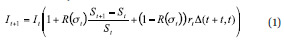where ?(t+1, t) denotes the time between date t and t+1 in the respective date-count convention. In principle, leveraged equity indexes and target volatility indexes are calculated according to formula (1), although they are quite different in terms of the way the size of the equity investment R(?t) is determined (as we will elaborate later) and also in terms of the rebalancing frequency: Leveraged indexes are typically rebalanced periodically (i.e., daily, weekly or monthly), whereas most target volatility indexes use a triggered rebalancing (i.e., rebalancing takes place when the actual weight of the equity investment in the index deviates by more than a predefined threshold from the desired weight R(?t)). Nevertheless, both index concepts can be analyzed within the same mathematical framework and can be improved by implementing an optimal risk-control mechanism in a similar way.

Based on the index methodology (1), the objective of our research is to answer the following question: Assume the underlying equity index St has an average yearly return ?, an average volatility ? and consequently a long-run Sharpe ratio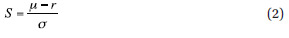with r denoting the average refinancing rate. Given these parameters of the underlying equity index, the question is whether it is possible to predict the long-run return and Sharpe ratio of the strategy index calculated according to formula (1) without knowing the entire path of the underlying equity index, i.e., only using the return and volatility of the underlying equity index and the response function R(?t).

We will show next that this is indeed possible for all existing types of strategy indexes based on the concept (1); in particular, leveraged indexes and target volatility indexes.

In a second step, we will use our understanding of the risk/ return characteristics of strategy indexes calculated according to the index formula (1) to derive optimally risk-controlled versions of leveraged indexes and target volatility indexes.

Analysis Of Existing Strategy Index Concepts
We start with an analysis of well-known investment strategies that are already available in the market in the form of strategy indexes and corresponding retail investment products: leveraged indexes and target-risk indexes.

Leveraged Indexes
The existing model of leveraged indexes has become quite popular in the ETF market in recent years (see EDHEC 2009). In fact, leveraged indexes are the simplest form of a strategy index using the index formula (1) in the sense that they are based on a constant response function. In the language of our model (1) we have (see NYSE Euronext 2008, Stoxx 2010):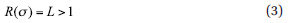where most leveraged ETFs use a constant leverage of L=2. In essence, the investment strategy (3) does not respond to equity volatility at all, which means it is not risk controlled in any way, and later in this article we will use the results derived for this type of leveraged index to show the advantages of strategies that are risk controlled.

Leveraged indexes have been widely criticized because their performance depends on the path of the underlying, as the index formula (1) suggests, and therefore their performance characteristics are very complex.

However, as we show in the mathematical derivation in Appendix 2 online (the same result has been shown in Despande 2009 and Giese 2010), in the long run, the performance of a leveraged index is governed by the law of great numbers, and therefore the long-run return g of a leveraged index is very accurately described by the following simple relationship: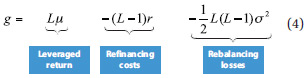In essence, the model equation (4) says that in the long run, the return of the leveraged index strategy is L times the return of the underlying equity index minus refinancing costs minus a term representing the adverse effect of rebalancing the portfolio on a continuous basis. In essence, this rebalancing loss comes from the well-known fact that the performance of leveraged indexes is path dependent.

This is a very important result, as it shows that leveraged ETFs on different underlying indexes and different leverage factors all exhibit the same long-run performance characteristics, which is essentially determined by three parameters:

1. The higher the yield ? of the underlying, the higher the more attractive leverage becomes.
2. The higher the average refinancing rate r, the higher the less attractive leverage is.
3. The higher the average realized volatility ? of the underlying, the higher the less attractive leverage becomes.

In other words, the complex path dependency of leveraged ETFs that is often cited as a main disadvantage of leveraged ETFs is absorbed in the long run into a simple rebalancing term that only depends on the average realized volatility of the underlying index during the investment period and the leverage factor of the index.

Based on the performance of leveraged indexes (4), we conclude the Sharpe ratio of a leveraged index is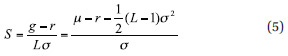The key observation from the Sharpe ratio (5) is that for a leveraged strategy (i.e., L>1), the Sharpe ratio turns out to be always smaller than the Sharpe ratio of the underlying index (2). The reasons for the deterioration in the Sharpe ratio are the aforementioned rebalancing losses, which reduce the outperformance of the leveraged index over the risk-free rate. Hence, even in strong bull markets where a leveraged index strongly outperforms a nonleveraged index in absolute terms, it will never outperform the nonleveraged index from a risk-adjusted perspective.

So far we have summarized theoretical results regarding leveraged indexes, which were derived in the online appendix based on stochastic calculus. This mathematical analysis is based on the model of equity returns following a normal distribution, in contrast to real-world equity returns, which are typically heavy tailed. Therefore, it is essential to verify the theoretical results by comparing them to real-world results. As a test case, we use the Euro Stoxx 50 Index and the EONIA money market rate as portfolio components.

Figure 1 compares the long-run returns of leveraged indexes for different degrees of leverage and compares them with the theoretical results obtained by the simple formula (4). The key observation is that the theoretical formula (2) provides a very accurate prediction of the long-run returns of leveraged indexes.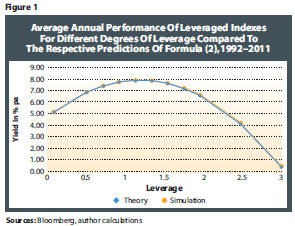In essence, the real-world backtests verify the fact that in the long run, the performance of leveraged indexes can be well understood according to the simple performance model (2) with a Sharpe ratio arrived at using formula (5).

To conclude, we have obtained the first key result of our analysis: Leveraged indexes without an embedded risk-control method (i.e., that do not respond to volatility in determining the size of the equity investment) have a Sharpe ratio that is always lower than the Sharpe ratio of the underlying equity index in the long run.

Target-Risk/Target-Volatility Indexes
Another common type of strategy index that has been launched by many index providers is the so-called target volatility scheme, which aims to keep the volatility of the strategy index It at a predefined constant target level T, which is achieved by using the following response function in the concept of the strategy index (1):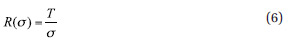In essence, determining the equity investment according to (6) means that the volatility of the strategy index will be the equity weight times equity volatility T/?* ?=T, i.e., it is constant. At first glance, the advantage of the target volatility strategy is that it keeps the market risk of the investment strategy at a constant level. In particular, it reduces the size of the equity investment in turbulent markets, which are typically characterized by increasing levels of market volatility, and thereby protects investors from more serious losses when markets are falling. On the other hand, when market volatility is very low (which is typically the case in markets that are booming), the size of the equity investment is increased, possibly even above 100 percent to take advantage of leveraged returns.

At a second and more theoretical glance, the advantages of the target-volatility index scheme are even more pronounced: The mathematical analysis summarized in Appendix 3 online shows that the long-run Sharpe ratio of the target-volatility index is always greater than the Sharpe ratio of the underlying equity index, as long as the target volatility level T is chosen below a certain threshold T< T*, which is greater than the long-run average volatility of the equity index, i.e., T *>?.

Economically speaking, the reason for the improvement in the Sharpe ratio is the fact that the target volatility strategy is taking equity risk at the right point in time in the investment cycle: It takes more equity risk when the risk/reward profile of equity markets is very favorable (i.e., when volatility is low), and takes less equity risk and therefore a larger size of fixed-income investment when the risk/reward profile of equity markets is less favorable.

Further, as we show in the online appendixes, the improvement of the Sharpe ratio of the target volatility strategy compared with its underlying blue-chip index is proportional to the volatility of the underlying equity index. The economic explanation is again obvious: When volatility is itself very volatile, then there is more opportunity to improve the Sharpe ratio compared with a plain equity investment by investing cyclically, i.e., taking more equity risk in phases of low volatility and less equity risk in phases of high volatility. Therefore, the more cyclical volatility is, the greater the improvement of the Sharpe ratio becomes.

The mathematical results of the risk/return characteristics of target volatility indexes derived in the online appendixes are summarized in Figure 2.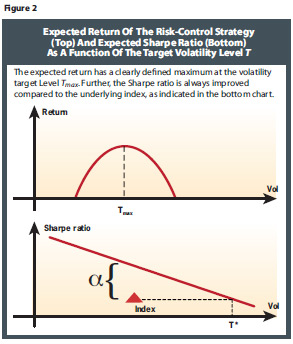Figure 2 shows that the expected return of the target volatility index increases in the target volatility level up to a maximum Tmax. In other words, it does not make sense to choose a target volatility level above Tmax, because above this threshold expected returns decrease, while the risk increases.

Figure 2 also demonstrates that the expected Sharpe ratio is a linearly decreasing function of the target volatility level that is located above the Sharpe ratio of the underlying equity index. In other words, a target volatility index with the target level chosen to equal the average level of volatility of the underlying equity index always has a better Sharpe ratio than the equity index in the long run.

Even for target volatility levels chosen above the average volatility of the underlying index, the Sharpe ratio is T*.

In essence, the theoretical results suggest that any target volatility strategy index based on any equity index creates alpha from a risk/return perspective, i.e., it has a better long-run Sharpe ratio, as long as the target volatility T*.

This is a very strong result, as it implies that investors in equity-based ETFs are always better off in the long run investing in the corresponding target volatility ETF instead of the pure equity ETF.

As for the leveraged indexes, it is important to verify these theoretical results by using real-world data. As a test case, we use the Euro Stoxx 50 Index and the EONIA money market rate as portfolio components. We compare the theoretical predictions for the expected return and expected Sharpe ratio detailed in the online appendixes and illustrated in Figure 2 to the actual simulation of the corresponding target volatility strategy using the index formulas (1) and (6). Figure 3 shows the expected returns and Sharpe ratios for a variety of target volatility levels T. The simulated values turn out to be very close to the predicted values across all target volatility levels for both expected returns and Sharpe ratios.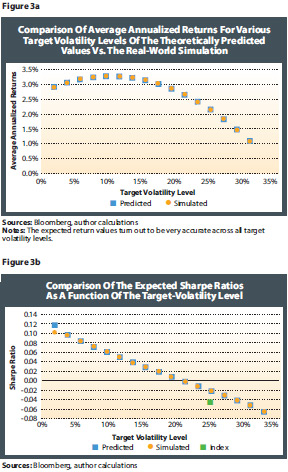In fact, the reason the heavy-tailed nature of equity return does not have a significant influence on our analysis is the results of our analysis in comparing long-term results, i.e., returns and Sharpe ratio over a period of several years. The influence of extreme adverse moves of equity markets, which appear occasionally due to the heavy tail of equity returns, get averaged out in the long run. In the short run, however, they can lead to significant differences between predicted and actual levels of expected returns and Sharpe ratios.

Optimal Risk-Control Mechanism
The existing strategies we have discussed so far—leveraged indexes and target volatility indexes—were derived heuristically but are not designed in an optimal way. As we show in this section, both for leveraged indexes and target volatility indexes, there is an optimal way to respond to volatility. Below we discuss the creation of optimal risk-control versions of both methodologies.

Optimally Risk-Controlled Leveraged Indexes
As we have seen in the previous section, leveraged indexes aim to enhance equity returns at the cost of lower Sharpe ratios. The main reason for this is the fact that existing leveraged indexes are not risk controlled.

The model for the expected return of leveraged indexes (5) shows that the rebalancing losses of leveraged indexes increase with equity volatility. Therefore, it makes sense to design leveraged indexes in a risk-controlled way, i.e., they should decrease the level of leverage when volatility goes up and vice versa.

Therefore, in Appendix 4 online, we derive an improved methodology that chooses the optimal way of adjusting the leverage factor (in other words, the size of the equity investment) to changes in volatility. The optimization shows that the optimal way of choosing the leverage factor is given by the following response function, determining the size of the equity investment of the leveraged index: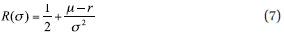where ?-r denotes the equity risk premium of the underlying equity index. Compared with the target volatility response function (6), the optimally risk-controlled leverage index (7) shows a stronger response to changes in volatility. In particular, it takes more equity risk than the target volatility index in markets of low volatility, as it is a pure return strategy: It does not try to meet a certain volatility level, but aims to maximize returns and takes into account the prevailing level of volatility, in contrast to existing leveraged indexes that are not risk controlled. In Appendix 4 online, we show that using the equity weight (7) creates a leveraged strategy index that has a higher expected return than the underlying equity index, i.e., it is an algorithmic alpha generator, which we will verify by numerical backtests below.

This is a very strong result, as it shows that incorporating a risk-control mechanism into the model framework of leveraged indexes creates strategy indexes that show a much stronger performance than existing strategy indexes in the long run.

Optimally Risk-Controlled Target Volatility Indexes
The bespoke target volatility strategy aims to keep the risk profile of the strategy index at a predefined volatility level, which can be achieved by choosing the equity investment according to the response function (6). However, this strategy does not optimize the long-run Sharpe ratio of the strategy index.

The optimal risk-control strategy chooses the equity investment in such a way that the Sharpe ratio of the resulting index is maximized under the condition that the expected volatility of the index remains at a predefined target T.

As we show in Appendix 5 online, the optimal target volatility index uses an equity investment that is very similar to the target volatility strategy (6), except that the equity investment is inversely proportional to the variance ?2 of ?, i.e.,with a proportionality factor given in Appendix 5 online. It is important to understand the economic difference between the target volatility strategy (6) and the optimal target volatility strategy (8): The target volatility strategy (6) is designed to keep the volatility of the investment scheme at the target level T at all times. However, the optimized response function (8) only targets the volatility level T on average and shows a stronger response to changes in volatility in the sense that in equity markets with relatively low levels of volatility, the equity investment is geared up such that investment portfolio is more volatile than average. On the other hand, when equity volatility is relatively high, the equity investment is reduced such that the investment portfolio is less volatile than average. To conclude, instead of keeping the volatility of the index portfolio at a constant level, the optimal risk strategy invests countercyclically: It takes more risk when equity markets are in a regime of low volatility but takes less risk when markets are at an above-average level of volatility. This countercyclical investment strategy achieves a better long-run Sharpe ratio than the pure target volatility strategy (6).

Numerical Results
To test the performance of the optimized risk-control mechanism presented above versus the nonoptimized methodologies, we use the Euro Stoxx 50 Index and the EONIA money market rate as portfolio components.

As a volatility measure, we will use a historical standard deviation of the returns of the past 60 trading days.

For estimating the return of the underlying equity index µ needed as input into (7), we use µ = the lower of either the annualized life-to-date return or the annualized return of the past 120 trading days of the index. The reason is that in the very long run, we expect µ to be (close to) constant. However, in the medium term, it is important to detect possible bear markets in the response function (7) to avoid situations where overly optimistic estimates of the underlying returns enter the investment scheme in the middle of a bear market.

We simulate a constant leverage strategy (3) with L=2, the target volatility strategy (6), the optimally risk-controlled leveraged strategy (7) (which we refer to as "optimal leverage") and the optimal target volatility strategy (8) (referred to as "optimal risk") using the Euro Stoxx 50 as an underlying equity investment from 1992 to 2011. For the target volatility strategy and the optimal risk strategy, we run two simulations—one with a target volatility level of 6 percent and one with 16 percent. The performance is plotted in Figure 4, whereas the risk/return matrix is shown in Figure 5. Figure 6 summarizes the key performance figures and risk/return characteristics.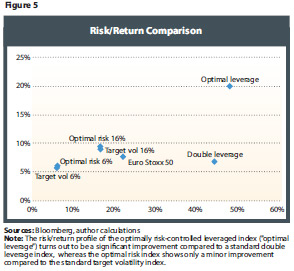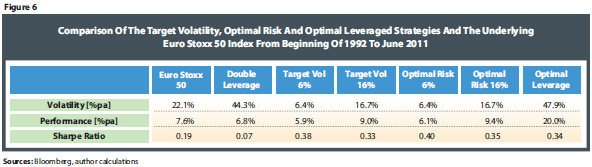We can draw two key observations from Figures 4 and 5. The first is that the target volatility strategy is very close to the optimal risk strategy in terms of risk/return profile and can therefore be considered as a very good proxy for the optimal risk investment scheme. Secondly, except for the constant leverage strategy, all schemes have a better risk/return profile than the underlying Euro Stoxx 50 Index, including the optimally risk-controlled leverage scheme ("optimal leverage"), which shows an impressive outperformance over 19 years.

The most popular investment scheme in the retail market (especially in the form of leveraged ETFs) is the double leverage approach, which shows a poor performance and very high levels of volatility in the long run. We therefore argue that investors are better off investing for the long term in either the optimal leverage strategy or the target volatility scheme, depending on their risk appetite.

Conclusion
We have derived a model for the risk/return profile of strategy indexes that combine an investment into an equity index and a money market investment in a rules-based way. We have shown that using a rebalancing function that responds to the volatility of the underlying equity market is crucial to achieve a favorable risk/return profile.

In the context of our model, we have seen that existing leveraged indexes are suboptimal from both a performance and Sharpe ratio perspective, as they are not risk controlled in any way, i.e., they use a constant equity proportion in their portfolio allocation.

We have shown that incorporating a risk-control mechanism into the framework of leveraged indexes in the form of a response function that responds adversely to volatility leads to significant improvements in terms of absolute performance and Sharpe ratio.

Regarding target volatility indexes, we have shown that their long-run Sharpe ratio is always better than the Sharpe ratio of the underlying equity index as long as the target volatility level is chosen within reasonable boundaries. Further, it is interesting to note that in practical simulations, we have seen that the existing target volatility strategy comes very close to the optimal risk strategy we have derived in this paper in terms of risk and performance profile. We therefore argue that from a practitioner's point of view, existing target volatility indexes respond to volatility in an (almost) risk/return optimal way.

It is important to mention that the mathematical results we have derived are independent of the underlying equity index and money market, i.e., they are robust across different markets and market regimes.

Finally, this paper provides a clear recommendation to investors in passive equity strategies: In the long run, investors in equity indexes (e.g., in the form of index-tracking ETFs or certificates) can clearly improve their long-run Sharpe ratio by shifting their investment to a corresponding target volatility index that offers comparable equity returns at lower levels of risk.

Further, we have seen that the existing methodology of leveraged indexes can be improved substantially by incorporating a risk-control mechanism. We therefore propose index providers adjust their methodologies for calculating leveraged indexes to incorporate the risk-control mechanisms outlined in this paper.

References
S&P 2010: Index mathematics, index methodologies, www.standardandpoors.com
Rules for the Leverage indexes, NYSE Euronext, April 2008
The EDHEC European ETF Survey 2009. May 2009. www.edhec-risk.com
M. Baxter, A. Rennie: Financial Calculus: An Introduction to Derivative Pricing, Cambridge University Press, 1996
M. Cheng, A. Madhavan: The Dynamics of Leveraged and Inverse-Exchange Traded Funds, Barclays Global Investors, May 2009
M. Despande, D. Mallick, R. Bhatia: "Understanding Ultrashort ETFs", Barclays Capital Special Report, 2009
G. Giese: "On the risk return profile of leveraged and inverse ETFs", in Journal of Asset Management, October 2010
L. Lu, J. Wang, G. Zhang: Long Term Performance of Leveraged ETFs, Working paper, available at http://ssrn.com/abstract=1344133, August 2009
R.C. Merton: "Optimum consumption and portfolio rules in a continuous time model", in Journal of Economic Theory, vol. 3, 1971
A.F. Perold and W.F. Sharpe: "Dynamic strategies for asset allocation", Financial Analysts Journal, January 1995

Endnote

Appendix 1: General Model Setup

To derive a theoretical understanding of strategy indices investing into an equity index and money market we rely on the well-known Brownian-motion model used in option pricing to describe the development of the equity index over time. To be precise, we assume a liquid underlying equity index St to model the equity investment of the strategy index that follows a stochastic process, i.e. with the growth rate u and the stochastic volatility ?t we have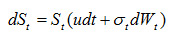(9)

Where Wt denotes a standard Wiener process. In essence, equation (9) states that the return of the equity index is driven by a continuous drift u and a standard normal random variable Wt with standard deviation ?t .

Thus, in the context of the stochastic process (9) the index formula (1) becomes:(10)

Or equivalently: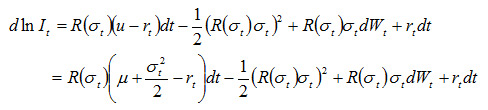(11)
With the observable growth rate of the underlying equity index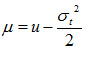In equation (10) dIt refers to infinitesimal changes of the investment value in time, whereas in real world applications dIt is typically implemented in the form of daily changes, based on a frequent re-balancing of the investment portfolio between equities and money market.

We are interested in the expected return g of the investment strategy, which can be derived by taking the expectation of equation (11):(12)
Where we used the average interest rate r = E[rt], the average volatility of the underlying St defined by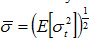(13)

the average equity investment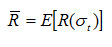and the average variance of the investment strategy It given by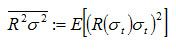(14).

From equation (12) we conclude that the expected return of the investment scheme is influenced by the response function R(.) in two opposite ways: First of all, the equity risk premium is scaled by the average value of the response function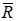and therefore higher values of the response function will increase equity related returns. At the same time, the expected return suffers from re-balancing losses that are driven by the average variance (14) of the investment scheme (as observed by Cheng and Madhavan 2009, Giese 2010). Since the scaling of the equity returns is linear in the response function and the re-balancing losses represent a quadratic effect in the re-balancing function there is an optimal trade-off between the two effects that depends on the volatility of the underlying equity markets. This is the crucial reason for implementing a re-balancing algorithm that responds to changes in the volatility of the underlying equity markets and will allow us to optimize the investment strategy (10) as shown below.

Moreover, the expected Sharpe-ratio of the investment strategy (10) reads: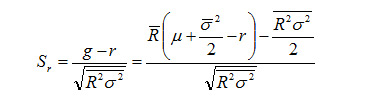(15)

Whereas the Sharpe-ratio of the underlying equity index reads(16)

Appendix 2: Leveraged Indices

Plugging the response function of leveraged indices R(?)=L into (12) yields the expected return of the leveraged strategy index: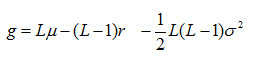Which is the same model equation as found in Depande 2009 and Giese 2010.

Appendix 3: Target Volatility Indices

With the response function of the target volatility strategy (6) the expected return (12) and Sharpe ratio (15) become: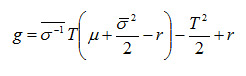(17)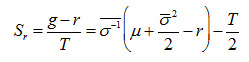(18)
With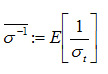The key question is under what circumstances the Sharpe-ratio of the target volatility strategy (17) is greater than the Sharpe-ratio of the underlying equity index (16), i.e.: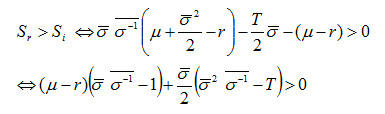(19)
Assuming a positive equity risk premium µ>r we conclude that equation (19) is certainly positive if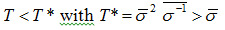Where we have used the fact that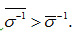.

This is a very strong results since it shows that irrespective of the underlying equity index and irrespective of the probability distribution of volatility the target volatility strategy always creates a better Sharpe-ratio than the underlying equity index in the long run as long as the volatility target is chosen below the threshold T*, which is greater than the average volatility of the underlying index.

It is also interesting to note from (19) that the improvement of the Sharpe-ratio is determined by the average inverse volatility, which is driven by the volatility of volatility as can be seen from the following second order approximation: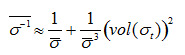Therefore, we argue that the volatility of volatility plays the role of an “opportunity parameter”, i.e. the more volatile equity volatility becomes, the higher the improvement in Sharpe-ratio compared to the underlying equity index is, because there is more opportunity to outperform the underlying index by responding to volatility. This result shows again how important it is to incorporate volatility into the response function.

Further, we observe that the expected return (17) is a quadratic function in the target volatility level T with a clearly defined maximal return at the target volatility levelWhich means using a higher target volatility level than Tmax is not reasonable. Further, the expected Sharpe-ratio (18) is a linear and decreasing function in the target volatility level T which lies above the expected return of the underlying index as indicated in Figure 2.

Appendix 4: Optimally Risk Controlled Leveraged Indices

We optimize the expected return (12) with respect to the response function R(.), i.e. we solve the variation equationTo optimize the expected return (12) we use a variation of the optimal response function, i.e.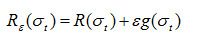With h(.) denoting the probability distribution of the volatility s, the optimality condition reads: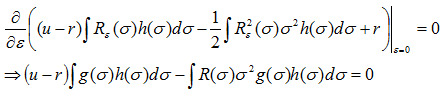Substituting g(.) for the delta distribution ?(?-?') and renaming ?' back to ? yields: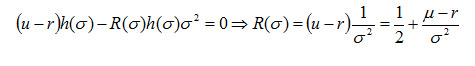The corresponding expected return of the optimally risk controlled leveraged index follows by plugging (7) into equation (12):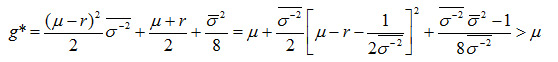(20)

with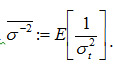From the right hand side of equation (20) we see that the expected return is always greater than the return of the underlying index m due that fact that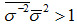, irrespective of the probability distribution of volatility and the average interest rate r.

This is a very strong result since it shows that in the long run a strategy index using the response function (7) in the investment strategy (10) always creates absolute outperformance over the underlying equity index, i.e. in the long run the optimally risk controlled leveraged index is an algorithmic alpha generator.

Further, the expected volatility of the optimal strategy reads: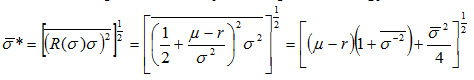(21)

Thus, the Sharpe-ratio becomes: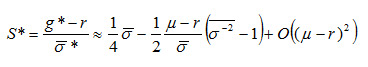(22)

The Sharpe-ratio (22) decreases in the equity risk premium m-r, which is not surprising because higher values of the equity risk premium will result in higher values of the response function (7) which increase volatility-driven re-balancing losses as explained above.

Appendix 5: Optimal Target Volatility Indices

As a next step we are looking for an optimal target volatility response function, i.e. we are
looking for the response function that maximizes the expected return for a given level of expected volatility T: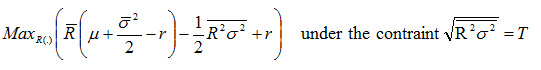(23)

In contrast to the target volatility strategy discussed above, the optimal risk strategy only targets a certain volatility level T on average, whereas the risk control function (6) is designed to keep the investment strategy at the desired volatility level at all times.

To optimize the expected return for a given level of expected volatility we can plug the constraint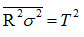(24)

into the expected return (12) and at the same time take into account this constraint via a Lagrange-parameter l in the following Lagrange-function: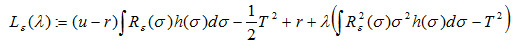The first order optimality condition reads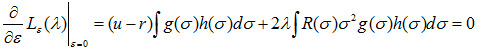Substituting g(.) for the delta distribution ?(?-?') and renaming ?' back to ? yields: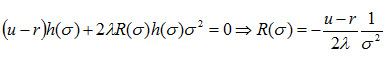(25)

To determine ? we use (25) in the constraint (24):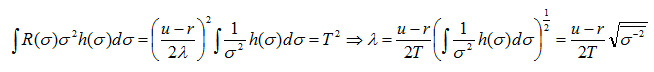With which the risk-return optimal response function (25) reads: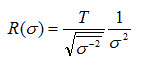(26)

The expected return of the optimal risk strategy index reads: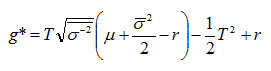(27)

The Sharpe-ratio becomes: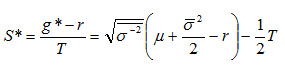(28)

The Sharpe-ratio (28) is always greater than the Sharpe-ratio of the underlying index and greater than the Sharpe-ratio of the target volatility index (18) because in general we have: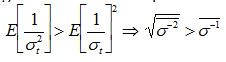This is a particularly strong result since it shows that the Sharpe-ratio of the target volatility index (which already improves the Sharpe-ratio of the underlying equity index as shown above) is even further improved, making it an ideal underlying for financial products that are designed to offer equity returns with the best possible risk controlling mechanism to investors. As for the target volatility scheme, the improvement in Sharpe-ratio compared to the underlying index is driven by the volatility of the volatility of the underlying index, since in the expected return (27) we have in second order approximation: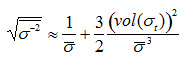It is interesting to compare the response function of the risk control strategy (6) to the optimal risk response function (26). The target volatility strategy is designed to keep the volatility of the investment scheme constantly at the target level T. However, the optimal risk response function (26) only targets the volatility level T on average and shows a stronger response to volatility in the sense that in equity markets with relatively low levels of volatility the equity investment is geared up such that investment portfolio is above average volatile. On the other hand, when equity volatility is relatively high the equity investment is reduced such that the investment portfolio is below average volatile. To conclude, instead of keeping the volatility of the portfolio at a constant level the optimal risk strategy invests counter-cyclically, i.e., it takes more risk when equity markets are in a regime of low volatility but takes less risk when markets are above average volatile.